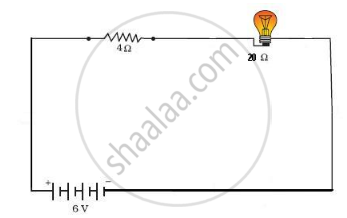Share

# An Electric Bulb of Resistance 20 ω and a Resistance Wire of 4 ω Are Connected in Series with a 6 V Battery. Draw the Circuit Diagram, and Calculate: (A) Total Resistance of the Circuit. (B) Current Through the Circuit. (C) Potential Difference Across the Electric Bulb. (D) Potential Difference Across the Resistance Wire. - CBSE Class 10 - Science

#### Question

An electric bulb of resistance 20 Ω and a resistance wire of 4 Ω are connected in series with a 6 V battery.
Draw the circuit diagram, and calculate:

(a) total resistance of the circuit.
(b) current through the circuit.
(c) potential difference across the electric bulb.
(d) potential difference across the resistance wire.

#### Solution(a) Total resistance, R = 20 Ω + 4 Ω = 24 Ω
(b) Current through the circuit,
I = V/R
I = 6/24
I = 0.25 A
(c) Potential difference across the electric bulb,
Vbulb = IRbulb
=0.25xx20
= 5 V
(d) Potential difference across the resistance wire,
Vwire = IRwire
= 0.25xx4
=  1 V

Is there an error in this question or solution?

#### Video TutorialsVIEW ALL 

Solution An Electric Bulb of Resistance 20 ω and a Resistance Wire of 4 ω Are Connected in Series with a 6 V Battery. Draw the Circuit Diagram, and Calculate: (A) Total Resistance of the Circuit. (B) Current Through the Circuit. (C) Potential Difference Across the Electric Bulb. (D) Potential Difference Across the Resistance Wire. Concept: Electric Current and Circuit.
S